# Overview of Chainer and Its Features

Mar. 23, 2016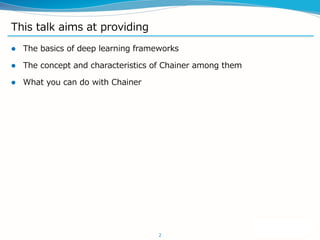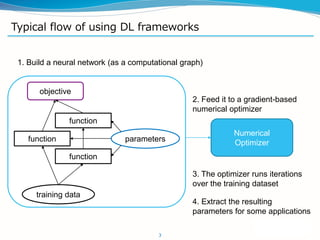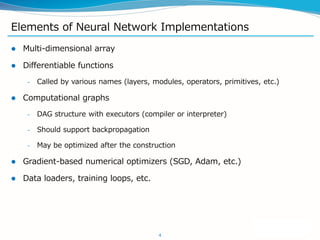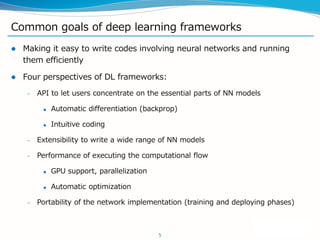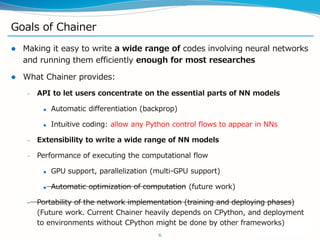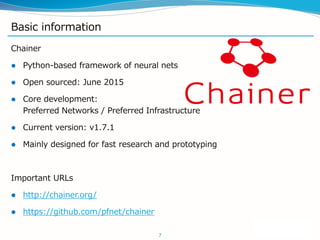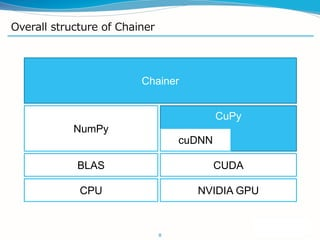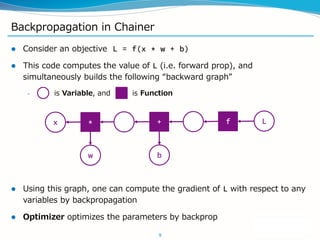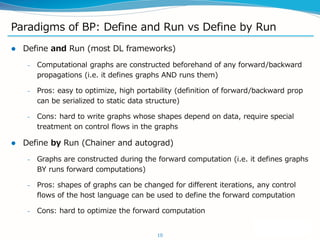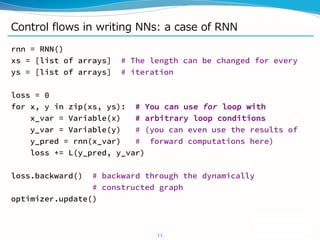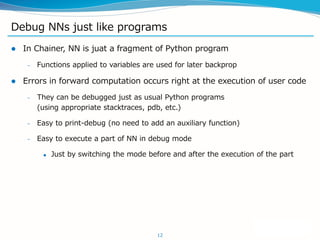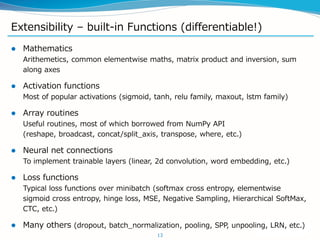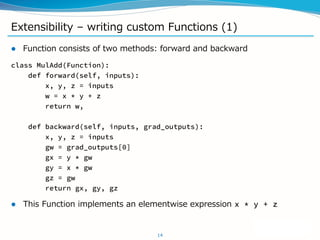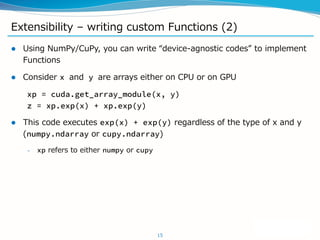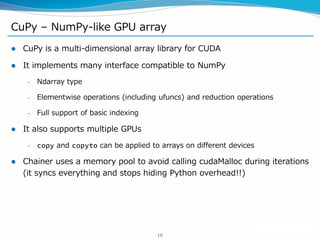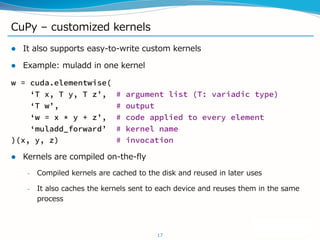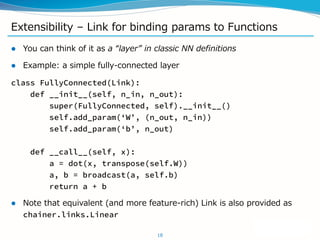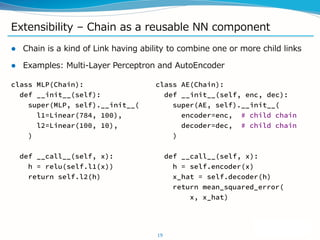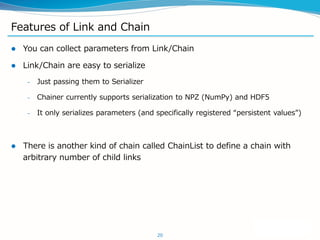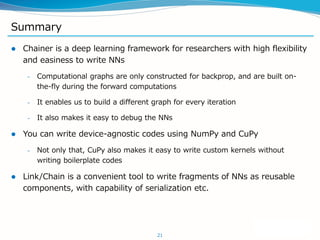1 of 21

### Overview of Chainer and Its Features

1. Overview of Chainer and Its Features Deep Learning Tokyo 2016 at Yahoo! JAPAN Seiya Tokui, Preferred Networks, Inc. Mar. 20, 2016
2. This talk aims at providing  The basics of deep learning frameworks  The concept and characteristics of Chainer among them  What you can do with Chainer 2
3. Typical flow of using DL frameworks 3 objective training data function function function parameters 1. Build a neural network (as a computational graph) 2. Feed it to a gradient-based numerical optimizer Numerical Optimizer 3. The optimizer runs iterations over the training dataset 4. Extract the resulting parameters for some applications
4. Elements of Neural Network Implementations  Multi-dimensional array  Differentiable functions – Called by various names (layers, modules, operators, primitives, etc.)  Computational graphs – DAG structure with executors (compiler or interpreter) – Should support backpropagation – May be optimized after the construction  Gradient-based numerical optimizers (SGD, Adam, etc.)  Data loaders, training loops, etc. 4
5. Common goals of deep learning frameworks  Making it easy to write codes involving neural networks and running them efficiently  Four perspectives of DL frameworks: – API to let users concentrate on the essential parts of NN models  Automatic differentiation (backprop)  Intuitive coding – Extensibility to write a wide range of NN models – Performance of executing the computational flow  GPU support, parallelization  Automatic optimization – Portability of the network implementation (training and deploying phases) 5
6. Goals of Chainer  Making it easy to write a wide range of codes involving neural networks and running them efficiently enough for most researches  What Chainer provides: – API to let users concentrate on the essential parts of NN models  Automatic differentiation (backprop)  Intuitive coding: allow any Python control flows to appear in NNs – Extensibility to write a wide range of NN models – Performance of executing the computational flow  GPU support, parallelization (multi-GPU support)  Automatic optimization of computation (future work) – Portability of the network implementation (training and deploying phases) (Future work. Current Chainer heavily depends on CPython, and deployment to environments without CPython might be done by other frameworks) 6
7. Basic information 7 Chainer  Python-based framework of neural nets  Open sourced: June 2015  Core development: Preferred Networks / Preferred Infrastructure  Current version: v1.7.1  Mainly designed for fast research and prototyping Important URLs  http://chainer.org/  https://github.com/pfnet/chainer
8. Overall structure of Chainer 8 CuPy CPU NVIDIA GPU CUDA cuDNN BLAS NumPy Chainer
9. Backpropagation in Chainer  Consider an objective L = f(x * w + b)  This code computes the value of L (i.e. forward prop), and simultaneously builds the following “backward graph” – is Variable, and is Function  Using this graph, one can compute the gradient of L with respect to any variables by backpropagation  Optimizer optimizes the parameters by backprop 9 f* +x w b L
10. Paradigms of BP: Define and Run vs Define by Run  Define and Run (most DL frameworks) – Computational graphs are constructed beforehand of any forward/backward propagations (i.e. it defines graphs AND runs them) – Pros: easy to optimize, high portability (definition of forward/backward prop can be serialized to static data structure) – Cons: hard to write graphs whose shapes depend on data, require special treatment on control flows in the graphs  Define by Run (Chainer and autograd) – Graphs are constructed during the forward computation (i.e. it defines graphs BY runs forward computations) – Pros: shapes of graphs can be changed for different iterations, any control flows of the host language can be used to define the forward computation – Cons: hard to optimize the forward computation 10
11. Control flows in writing NNs: a case of RNN rnn = RNN() xs = [list of arrays] # The length can be changed for every ys = [list of arrays] # iteration loss = 0 for x, y in zip(xs, ys): # You can use for loop with x_var = Variable(x) # arbitrary loop conditions y_var = Variable(y) # (you can even use the results of y_pred = rnn(x_var) # forward computations here) loss += L(y_pred, y_var) loss.backward() # backward through the dynamically # constructed graph optimizer.update() 11
12. Debug NNs just like programs  In Chainer, NN is juat a fragment of Python program – Functions applied to variables are used for later backprop  Errors in forward computation occurs right at the execution of user code – They can be debugged just as usual Python programs (using appropriate stacktraces, pdb, etc.) – Easy to print-debug (no need to add an auxiliary function) – Easy to execute a part of NN in debug mode  Just by switching the mode before and after the execution of the part 12
13. Extensibility – built-in Functions (differentiable!)  Mathematics Arithemetics, common elementwise maths, matrix product and inversion, sum along axes  Activation functions Most of popular activations (sigmoid, tanh, relu family, maxout, lstm family)  Array routines Useful routines, most of which borrowed from NumPy API (reshape, broadcast, concat/split_axis, transpose, where, etc.)  Neural net connections To implement trainable layers (linear, 2d convolution, word embedding, etc.)  Loss functions Typical loss functions over minibatch (softmax cross entropy, elementwise sigmoid cross entropy, hinge loss, MSE, Negative Sampling, Hierarchical SoftMax, CTC, etc.)  Many others (dropout, batch_normalization, pooling, SPP, unpooling, LRN, etc.) 13
14. Extensibility – writing custom Functions (1)  Function consists of two methods: forward and backward class MulAdd(Function): def forward(self, inputs): x, y, z = inputs w = x * y + z return w, def backward(self, inputs, grad_outputs): x, y, z = inputs gw = grad_outputs gx = y * gw gy = x * gw gz = gw return gx, gy, gz  This Function implements an elementwise expression x * y + z 14
15. Extensibility – writing custom Functions (2)  Using NumPy/CuPy, you can write “device-agnostic codes” to implement Functions  Consider x and y are arrays either on CPU or on GPU xp = cuda.get_array_module(x, y) z = xp.exp(x) + xp.exp(y)  This code executes exp(x) + exp(y) regardless of the type of x and y (numpy.ndarray or cupy.ndarray) – xp refers to either numpy or cupy 15
16. CuPy – NumPy-like GPU array  CuPy is a multi-dimensional array library for CUDA  It implements many interface compatible to NumPy – Ndarray type – Elementwise operations (including ufuncs) and reduction operations – Full support of basic indexing  It also supports multiple GPUs – copy and copyto can be applied to arrays on different devices  Chainer uses a memory pool to avoid calling cudaMalloc during iterations (it syncs everything and stops hiding Python overhead!!) 16
17. CuPy – customized kernels  It also supports easy-to-write custom kernels  Example: muladd in one kernel w = cuda.elementwise( ‘T x, T y, T z’, # argument list (T: variadic type) ‘T w’, # output ‘w = x * y + z’, # code applied to every element ‘muladd_forward’ # kernel name )(x, y, z) # invocation  Kernels are compiled on-the-fly – Compiled kernels are cached to the disk and reused in later uses – It also caches the kernels sent to each device and reuses them in the same process 17
18. Extensibility – Link for binding params to Functions  You can think of it as a “layer” in classic NN definitions  Example: a simple fully-connected layer class FullyConnected(Link): def __init__(self, n_in, n_out): super(FullyConnected, self).__init__() self.add_param(‘W’, (n_out, n_in)) self.add_param(‘b’, n_out) def __call__(self, x): a = dot(x, transpose(self.W)) a, b = broadcast(a, self.b) return a + b  Note that equivalent (and more feature-rich) Link is also provided as chainer.links.Linear 18
19. Extensibility – Chain as a reusable NN component  Chain is a kind of Link having ability to combine one or more child links  Examples: Multi-Layer Perceptron and AutoEncoder 19 class MLP(Chain): def __init__(self): super(MLP, self).__init__( l1=Linear(784, 100), l2=Linear(100, 10), ) def __call__(self, x): h = relu(self.l1(x)) return self.l2(h) class AE(Chain): def __init__(self, enc, dec): super(AE, self).__init__( encoder=enc, # child chain decoder=dec, # child chain ) def __call__(self, x): h = self.encoder(x) x_hat = self.decoder(h) return mean_squared_error( x, x_hat)
20. Features of Link and Chain  You can collect parameters from Link/Chain  Link/Chain are easy to serialize – Just passing them to Serializer – Chainer currently supports serialization to NPZ (NumPy) and HDF5 – It only serializes parameters (and specifically registered “persistent values”)  There is another kind of chain called ChainList to define a chain with arbitrary number of child links 20
21. Summary  Chainer is a deep learning framework for researchers with high flexibility and easiness to write NNs – Computational graphs are only constructed for backprop, and are built on- the-fly during the forward computations – It enables us to build a different graph for every iteration – It also makes it easy to debug the NNs  You can write device-agnostic codes using NumPy and CuPy – Not only that, CuPy also makes it easy to write custom kernels without writing boilerplate codes  Link/Chain is a convenient tool to write fragments of NNs as reusable components, with capability of serialization etc. 21